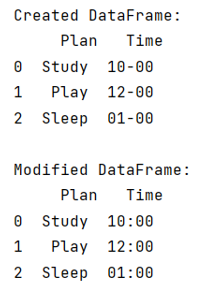# How to replace text in a string column of a Pandas DataFrame?

Given a Pandas DataFrame, we have to replace text in a string column. By Pranit Sharma Last updated : September 22, 2023

Pandas is a special tool that allows us to perform complex manipulations of data effectively and efficiently. Inside pandas, we mostly deal with a dataset in the form of DataFrame. DataFrames are 2-dimensional data structures in pandas. DataFrames consist of rows, columns, and the data. The Data inside the DataFrame can be of any type.

## Problem statement

Given a Pandas DataFrame, we have to replace text in a string column. Suppose that the type of data inside the DataFrame is of string type and we need to replace some string with some other string.

## Replacing text in a string column of a Pandas DataFrame

For this purpose, we will use pandas.Series.str.replace() method by passing the old and new strings i.e., the values to be replaced and to be replaced with. It replaces each occurrence of pattern/regex in the Series/Index.

The syntax of the replace() method is:

```Series.str.replace(pat, repl, n=-1, case=None, flags=0, regex=False)
```
Note

To work with pandas, we need to import pandas package first, below is the syntax:

```import pandas as pd
```

Let us understand with the help of an example,

## Python program to replace text in a string column of a Pandas DataFrame

```# Importing pandas package
import pandas as pd

# Importing numpy package
import numpy as np

# Creating a dictionary
d = {
'Plan':['Study','Play','Sleep'],
'Time':['10-00','12-00','01-00']
}

# Creating a DataFrame
df = pd.DataFrame(d)

# Display DataFrame
print("Created DataFrame:\n",df,"\n")

# Replacing the '-' with ':' in time column
df['Time'] = df['Time'].replace('-',':',regex=True)

# Dispay modified DataFrame
print("Modified DataFrame:\n",df)
```

### Output

The output of the above program is: